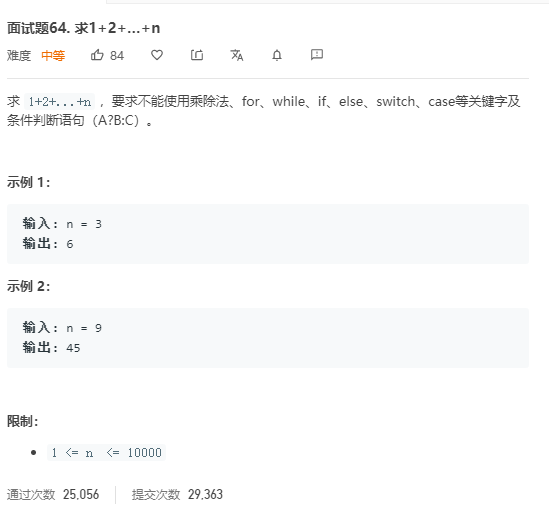### 题目描述#### 方法一

class Solution:
def sumNums(self, n: int) -> int:
return n!=0 and n + self.sumNums(n-1)


### Note:

if(A && B) // 若 A 为 false ，则 B 的判断不会执行（即短路），直接判定 A && B 为 false
if(A || B) // 若 A 为 true ，则 B 的判断不会执行（即短路），直接判定 A || B 为 true

• 本题需要实现当n=0时终止递归的需求 即
n!=0 and n+self.Nums(n-1)实现